IMO Shortlist 2009 problem N6

Kvaliteta:
Avg: 0,0
Težina:
Avg: 8,0
Let$k$ be a positive integer. Show that if there exists a sequence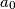$a_0$,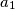$a_1$, ... of integers satisfying the condition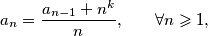then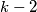$k-2$ is divisible by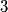$3$.

Proposed by Turkey
Izvor: Međunarodna matematička olimpijada, shortlist 2009